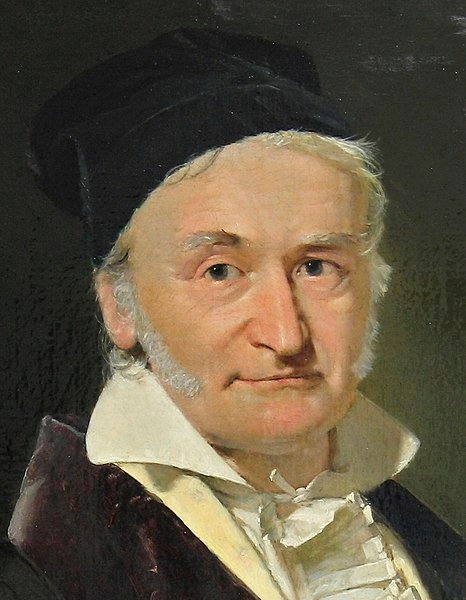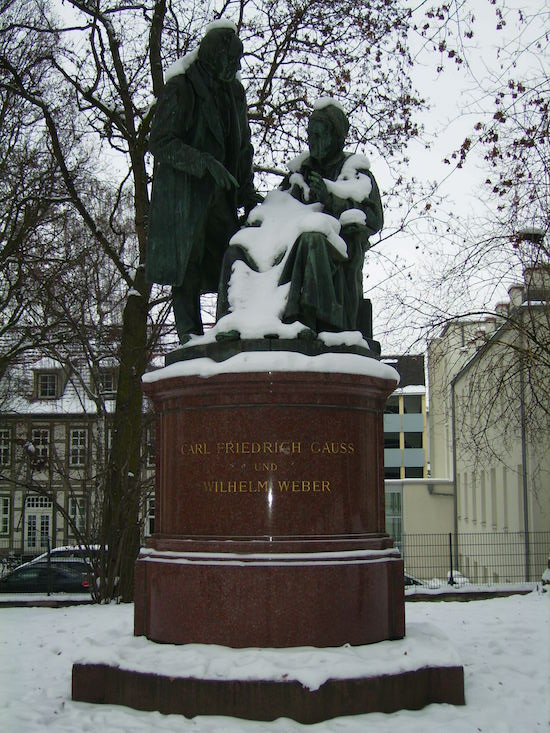## Happy Birthday, Carl Friedrich Gauss

#####Emily Ediger April 30, 2019

German mathematician Carl Friedrich Gauss was so proficient at mathematics that he is considered to be one of the greatest mathematicians of all time. He was also talented in other subjects, including cartography, physics, and planetary astronomy. Today, Gauss even has an entire unit system as his namesake!

### Gauss’ Strength in Calculations

Carl Friedrich Gauss was born on April 30, 1777, in Brunswick, Germany. As a boy, Gauss was gifted in language, but he also showed aptitude in mathematics and could perform elaborate calculations in his head. Because of his impressive skills, Gauss received financial support from the Duke of Brunswick to pursue his education — first locally and then at university.A painting of Carl Friedrich Gauss. Image in the public domain, via Wikimedia Commons.

Gauss attended what is now known as the Technical University of Braunschweig, as well as the University of Göttingen, where he studied mathematics. In 1797, Gauss completed his doctoral dissertation on the discussion of the fundamental theorem of algebra at the University of Helmstedt.

In his dissertation, Gauss proved the fundamental theorem of algebra, which states that every polynomial equation with real or complex coefficients has as many complex-valued roots as its degree. Gauss also discovered that a regular polygon (with 17 sides) could be constructed with a straight edge (no increment markings) and compass alone. This represented a much deeper understanding of the roots of polynomial equations.

### Carl Friedrich Gauss: A Mathematician with Many Talents

In 1801, Carl Friedrich Gauss produced a text that secured his reputation as one of the greatest mathematicians of all time. Disquisitiones Arithmeticae was the first systematic textbook on algebraic number theory.

Gauss also wrote about the orbit of the asteroid Ceres. First discovered in 1801, Ceres was elusive to many scientists who had attempted to calculate its path and predict where it would appear next. Gauss was successful with his calculations, establishing fast Fourier transform and the method of least squares in the process.

Gauss’ capability with calculations lended well to other areas, including cartography and the mathematical theory of map construction. In the 1830s, Gauss also explored terrestrial magnetism and joined the first survey of Earth’s magnetic field.At the University of Göttingen, the Gauss–Weber Monument commemorates Carl Friedrich Gauss and physicist Wilhelm Weber.

Though Gauss’ talents spread into many different areas, he produced few published works and was not a fan of teaching. There are many speculations as to why Gauss kept a lot of his work under wraps, including his reputation as a perfectionist. Many of his ideas and theories were not discovered until his death in 1855.

Gauss certainly left an indelible mark on the fields of mathematics and science. He was awarded the Lalande Prize and the Copley Medal in recognition of his achievements. The Carl Friedrich Gauss Prize for Applications of Mathematics is one of the highest honors in mathematics today.

The most common bell curve in statistics, the normal distribution, was named the Gaussian distribution in remembrance of Gauss. There is also an entire centimeter-gram-second (cgs) unit system for electromagnetics known as Gaussian units.

Let’s all wish Carl Friedrich Gauss a happy birthday!